Courses

# RD Sharma Solutions Ex-14.2, Quadrilaterals, Class 9, Maths Class 9 Notes | EduRev

## Class 9 : RD Sharma Solutions Ex-14.2, Quadrilaterals, Class 9, Maths Class 9 Notes | EduRev

The document RD Sharma Solutions Ex-14.2, Quadrilaterals, Class 9, Maths Class 9 Notes | EduRev is a part of the Class 9 Course RD Sharma Solutions for Class 9 Mathematics.
All you need of Class 9 at this link: Class 9

Q.1. Two opposite angles of a parallelogram are (3x-2)0 and (50-x)0. Find the measure of each angle of the parallelogram.

Solution:

We know that,

Opposite sides of a parallelogram are equal.

(3x-2)0 = (50-x)0

⇒ 3x + x = 50 + 2

⇒ 4x = 52

⇒ x = 130

Therefore, (3x-2)0 = (3*13-2) = 370

(50-x)0 = (50-13) = 370

Adjacent angles of a parallelogram are supplementary.

∴ x+37 = 1800

∴ x = 1800−370 = 1430

Hence, four angles are : 370, 1430, 370, 1430.

Q.2. If an angle of a parallelogram is two-third of its adjacent angle, find the angles of the parallelogram.

Solution:

Let the measure of the angle be x.

Therefore, the measure of the angle adjacent is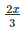We know that the adjacent angle of a parallelogram is supplementary.

Hence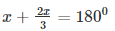2x + 3x = 5400

⇒ 5x = 5400

⇒ x = 1080

⇒ x + 1080 = 1800

⇒ x = 1800 – 1080 = 720

⇒ x = 720

Hence, four angles are 1800, 720, 1800, 720

Q.3. Find the measure of all the angles of a parallelogram, if one angle is 240 less than twice the smallest angle.

Solution:

x + 2x – 24 = 1800

⇒ 3x – 24 = 1800

⇒ 3x = 1080 + 24

⇒ 3x = 2040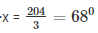⇒ x = 680

⇒ 2x – 240 = 2*680 – 240 = 1120

Hence, four angles are 680, 1120, 680, 1120.

Q.4. The perimeter of a parallelogram is 22cm. If the longer side measures 6.5cm what is the measure of the shorter side?

Solution:

Let the shorter side be ‘x’.

Therefore, perimeter = x + 6.5 + 6.5 + x       [Sum of all sides]

22 = 2 (x + 6.5)

11 = x + 6.5

⇒ x = 11 – 6.5 = 4.5cm

Therefore, shorter side = 4.5cm

Q.5. In a parallelogram ABCD, ∠D = 1350. Determine the measures of ∠Aand∠B.

Solution:

In a parallelogram ABCD

So, ∠D+∠C = 1800

∠C = 1800−1350

∠C = 450

In a parallelogram opposite sides are equal.

∠A =∠C = 450

∠B = ∠D = 1350

Q. 6. ABCD is a parallelogram in which ∠A = 700. Compute ∠B,∠Cand∠D.

Solution:

In a parallelogram ABCD

∠A = 700

∠A+∠B = 1800                 [ Since, adjacent angles are supplementary ]

700+∠B = 1800                      [∵∠ A = 70°]

∠B = 1800−700

∠B =1100

In a parallelogram opposite sides are equal.

∠A = ∠C = 700

∠B = ∠D = 1100

Q.7. In Figure 14.34, ABCD is a parallelogram in which ∠A = 600. If the bisectors of ∠A,and∠B meet at P, prove that AD = DP, PC = BC and DC = 2AD.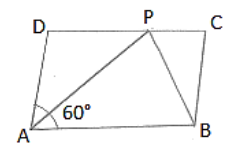Solution:

AP bisects ∠A

Then, ∠DAP = ∠PAB = 300

Then, ∠A+∠B = 1800

∠B+600 = 1800

∠B = 1800−600

∠B = 1200

BP bisects ∠B

Then, ∠PBA = ∠PBC = 300

∠PAB = ∠APD = 300    [Alternate interior angles]

Therefore, AD = DP       [Sides opposite to equal angles are in equal length]

Similarly

∠PBA = ∠BPC = 600  [Alternate interior angles]

Therefore, PC = BC

DC = DP + PC

DC = AD + BC   [Since, DP = AD and PC = BC]

DC = 2AD          [Since, AD = BC, opposite sides of a parallelogram are equal]

Q.8. In figure 14.35, ABCD is a parallelogram in which ∠DAB = 750 and ∠DBC = 600. Compute ∠CDB, and ∠ADB.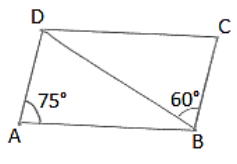Solution:

∠CBD = ∠ABD = 600     [Alternate interior angle. AD║BC and BD is the transversal]

In ∠BDC

∠CBD+∠C+∠CDB = 1800     [Angle sum property]

⇒ 600+750+∠CDB =1800

⇒ ∠CDB = 1800−(600+750)

⇒ ∠CDB = 450

Hence, ∠CDB = 450,∠ADB = 600

Q. 9. In figure 14.36, ABCD is a parallelogram and E is the mid-point of side BC. If DE and AB when produced meet at F, prove that AF = 2AB.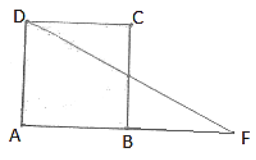Solution:

In ΔBEFandΔCED

∠BEF = ∠CED      [Verified opposite angle]

BE = CE                [Since, E is the mid-point of BC]

∠EBF = ∠ECD       [Since, Alternate interior angles are equal]

∴ ΔBEF ≅ ΔCED    [ASA congruence]

∴ BF = CD[CPCT]

AF = AB + AF

AF = AB + AB

AF = 2AB.

Hence proved.

Q.10. Which of the following statements are true (T) and which are false (F)?

(i) In a parallelogram, the diagonals are equal.

(ii) In a parallelogram, the diagonals bisect each other.

(iii) In a parallelogram, the diagonals intersect each other at right angles.

(iv) In any quadrilateral, if a pair of opposite sides is equal, it is a parallelogram.

(v) If all the angles of a quadrilateral are equal, it is a parallelogram.

(vi) If three sides of a quadrilateral are equal, it is a parallelogram.

(vii) If three angles of a quadrilateral are equal, it is a parallelogram.

(viii) If all the sides of a quadrilateral are equal, it is a parallelogram.

Solution:

(i) False

(ii) True

(iii) False

(iv) False

(v) True

(vi) False

(vii) False

(viii) True

Offer running on EduRev: Apply code STAYHOME200 to get INR 200 off on our premium plan EduRev Infinity!

91 docs

,

,

,

,

,

,

,

,

,

,

,

,

,

,

,

,

,

,

,

,

,

,

,

,

,

,

,

,

,

,